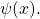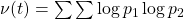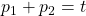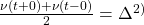# The Goldbach problem (II)

Aldo Peretti
Notes on Number Theory and Discrete Mathematics, ISSN 1310-5132
Volume 2, 1996, Number 1, Pages 53—68

## Details

### Authors and affiliations

Aldo Peretti
Rodriguez Pena 640, (1020) Buenos Aires, Argentina

### Abstract

It is studied in first place the function, where the sum is extended for all prime numbers pi such that. Are proved formulas (14) and(26), which express its value in terms of Chebyshev’s functionIn this way is obtained formula (38), that gives the asymptotic value of s(t) with a new ”singular” series which runs through the zeros of the Zeta function, but that at present can not be evaluated in a sufficiently accurate form. In second place, for the function(with), already considered by Hardy-Littlewood in ”Partitio Numerorum III” (P.N.III), is proved the exact formulawheresecond difference;andare the direct and inverse Laplace transforms;The circle method applied in P.N.III is equivalent to determinethrough the complex inversion formula along a Bromwich contour. But it is evident that is much preferable to employ tables of direct and inverse transforms because the functions in­volved are elementary; because is obtained an exact expression for the remainder, and because all the calculus is by far more simple. One arrive thus to the inconditional formula (130), which very closely resembles the famous conjecture A of P.N.III.

### References

1. E. Landau – Vorlesungen Uber Zaklentheorie – Chelsea Publishing Co. Theorem 452 – VII part, Chap. 10 § 2, p. 116.
2. D. Wolke. On the explicit formula of Riemann-von Mangoldt II – J. London Math. Soc. (2) 28 (1983) N° 3 p. 406-416
3. G. Hardy and J. Littlewood: Some problems in “Partitio Numerorum” III – On the expression of a number as a sum of primes. Acta Math. (1922) p. 1-70
4. Gradshteyn and Ryzhyk – Table of integrals, series and products – Academic Press (1965) p. 66 formula 2.153.
5. Idem (1), part V, Chap. 6, p. 218.
6. G. Can’. Formulas and theorems in pure Mathematics, p. 551. Chelsea edition, 1970
7. N. Nielsen – Handbuch der Gamma Funktion, p.71.
8. A. Peretti: The method of the Laplace transform compared with the circle method. Bull. Numb. Theory, Vol. X, Dec. 1986, N° 3 p.2-61.

## Cite this paper

Peretti, A. (1996). The Goldbach problem (II). Notes on Number Theory and Discrete Mathematics, 2(1), 53-68.